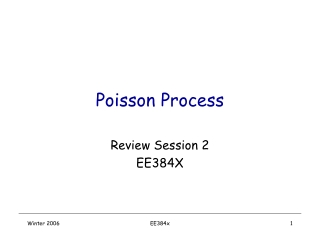DownloadDownload PresentationPoisson Process

# Poisson Process

Télécharger la présentation## Poisson Process

- - - - - - - - - - - - - - - - - - - - - - - - - - - E N D - - - - - - - - - - - - - - - - - - - - - - - - - - -
##### Presentation Transcript

1. Poisson Process Review Session 2 EE384X EE384x

2. Point Processes • Supermarket model : customers arrive (randomly), get served, leave the store • Need to model the arrival and departure processes Server Queue Departure Process Arrival Process EE384x

3. What does Poisson Process model? • Start time of Phone calls in Palo Alto • Session initiation times (ftp/web servers) • Number of radioactive emissions (or photons) • Fusing of light bulbs, number of accidents • Historically, used to model packets (massages) arriving at a network switch • (In Kleinrock’s PhD thesis, MIT 1964) EE384x

4. Properties • Say there has been 100 calls in an hour in Palo Alto • We expect that : • The start time of each call is independent of the others • The start time of each call is uniformly distributed over the one hour • The probability of getting two calls at exactly the same time is zero • Poisson Process has the above properties EE384x

5. Notation 0 EE384x

6. Notation • A(t) : Number of points in (0,t] • A(s,t) : Number of points in (s,t] • Arrival points : • Inter-arrival times: EE384x

7. Poisson Process- Definition • A(0)=0 and each jump is of unit magnitude • Number of arrivals in disjoint intervals are independent • For any the random variables are independent. • Number of arrivals in any interval of length tis distributed Poisson(lt) EE384x

8. Basic Properties EE384x

9. Stationary Increments • The number of arrivals in (t,t+t] does not depend on t EE384x

10. Orderliness • The probability of two or more arrivals in an interval of lengtht gets small as • Arrivals occur “one at a time” EE384x

11. Poisson Rate • Probability of one arrival in a short interval is (approx) proportional to interval length • Poisson process is like a continuous version of Bernoulli IID EE384x

13. Inter-arrival times • Inter-arrival times are Exponential(l) and independent of each other 0 EE384x

14. merge Merging two Poisson processes • Merging two independent Poisson processes with rates l1 and l2 creates a Poisson process with rate l1+l2 • A(0)=A1(0)+A2(0)=0 • Number of arrivals in disjoint intervals are independent • Sum of two independent Poisson rv is Poisson EE384x

15. Sum of two Poisson rv • Characteristic function: • So EE384x

16. Split Splitting a Poisson process :Poisson process with rate l • For each point toss a coin (with bias p): • With probability p the point goes to A1(t) • With probability 1-p the point goes to A2(t) • A1(t) and A2(t) are two independent Poisson processes with rates EE384x

17. proof • Define A1(t) and A2(t) such that: • A1(0)=0 A2(0)=0 • Number of points in disjoint intervals are independent for A1(t) and A2(t) • They depend on number of points in disjoint intervals of A(t) • Need to show that number of points of A1 and A2 in an interval of size tare independent Poisson(l1t) and Poisson(l2t) EE384x

18. Dividing a Poisson rv EE384x

19. Dividing a Poisson rv (cont) • So EE384x

20. Uniformity of arrival times • Given that there are n points in [0,t], the unordered arrival times are uniformly distributed and independent of each other. Unordered variables 0 Ordered variables EE384x

21. Single arrival case 0 EE384x

22. General case • It is the n order statistics of uniform distribution. EE384x